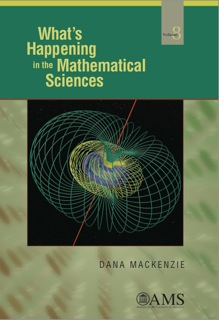Select Page

# WHIMS Volume 8What’s Happening in the Mathematical Sciences, Volume 8 covers the years from 2008 to 2010. Below is an annotated table of contents.

1. Accounting for Taste.

Netflix offered a million-dollar prize to develop a computer algorithm that could predict the tastes of Netflix customers 10 percent more accurately than their existing method. The results upset the conventional wisdom in the field of machine learning and demonstrated the power of “crowdsourcing.”

Branch of mathematics: Computer science.

2. A Brave New Symplectic World.

Thirty years ago, Alan Weinstein conjectured that certain kinds of dynamical systems with two degrees of freedom, such as a pendulum that is free to stretch as well as swing, always have periodic (repeating) solutions. Now “symplectic camels” and a “crazy” homology theory based on the equations for a magnetic monopole confirm that periodic orbits exist. (Magnetic monopoles still don’t.)

Branch of mathematics: Dynamical systems, geometry.

3. Mathematics and the Financial Crisis.

The credit crisis of 2007 and 2008 started one of the most serious recessions since World War II. Some observers blamed the crisis on “quants” (quantitative analysts). This chapter sorts fact from fiction and explains what math did, and didn’t, have to do with the financial meltdown. Moral: A mathematical model is only as good as its assumptions!

Branch of mathematics: Financial engineering, differential equations.

4. The Ultimate Billiard Shot.

In a weird version of billiards played on an infinite table, mathematicians prove the existence of “trick shots” that sink the ball into a pocket at infinity.

Branch of mathematics: Dynamical systems.

5. SimPatient.

This chapter looks at the growing role of mathematical models in public health policy, focusing on the recommendation of the U.S. Preventive Services Task Force in 2009 that women between age 40 and 49 should no longer be counseled to have yearly mammograms.

Branch of mathematics: Mathematical biology, applied mathematics.

6. Instant Randomness.

Markov Chain Monte Carlo (MCMC) methods are a powerful technique for simulating random processes, such as the alignment of electron spins in a ferromagnet (the Ising model). The technique depends on a phenomenon called “rapid mixing,” in which non-random initial guesses rapidly converge to random samples. Rapid mixing is now confirmed for the Ising model and certain other stochastic processes.

Branch of mathematics: Statistical physics, probability and statistics.

7. In Search of Quantum Chaos

A classical billiard ball, on a table with curved, “dispersing” sides, travels on a chaotic trajectory that essentially randomizes the ball’s position over the long term. However, at low energies, quantum billiard balls are not chaotic. In a tour de force combining pure number theory with physics, mathematicians proved that quantum chaos does emerge at high energies.

Branch of mathematics: Number theory, mathematical physics.

8. 3-D Surprises

Even in the twenty-first century, mathematics can still reveal new phenomena in ordinary three-dimensional space. Item 1: A stunningly efficient way to fit the most tetrahedra (triangular pyramids) into the least possible space. Item 2: The Gömböc, a homogeneous body that automatically rights (and “wrongs”) itself.

Branch of mathematics: Geometry.

9. As One Heroic Age Ends, a New One Begins

John Milnor’s discovery of 7-dimensional “exotic spheres” in 1956 launched a new era of high-dimensional topology. Heroic as his work was, it failed to resolve the number of exotic spheres in dimensions 14, 30, 62, etc. (all dimensions two less than a power of 2). After half a century, topologists finally managed to end the ambiguity.

Branch of mathematics: Topology.

Cover picture (foreground): The Hopf fibration arises from a topologically non-trivial way to map a 3-dimensional sphere (or “hypersphere”) into the ordinary 2-dimensional sphere. Maps of this type help topologists understand the structure of exotic spheres and other high-dimensional spaces. (See Chapter 9.)

Cover picture (background): The standard contact form in 3-dimensional space is illustrated as a field of planes. (See Chapter 2.)